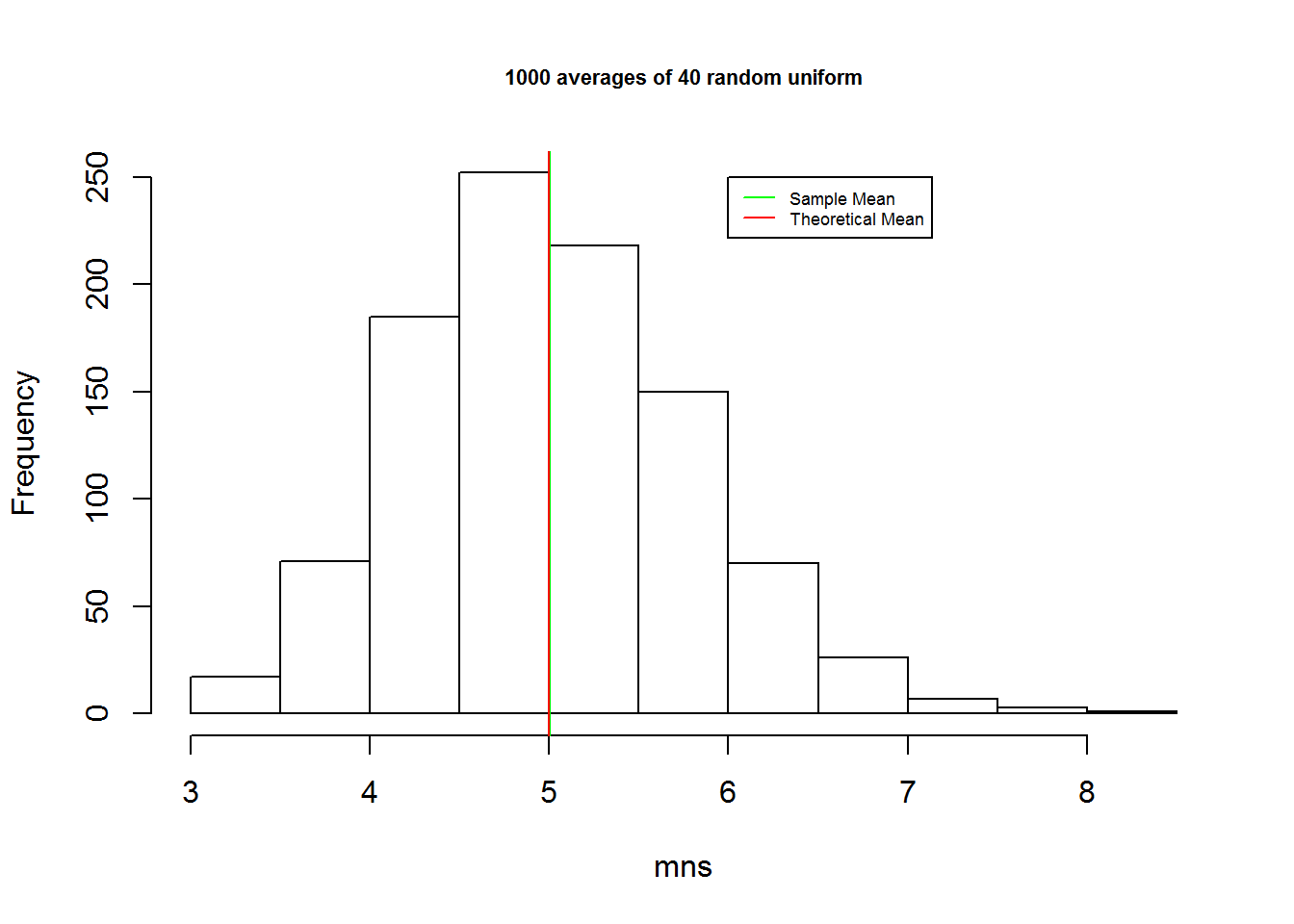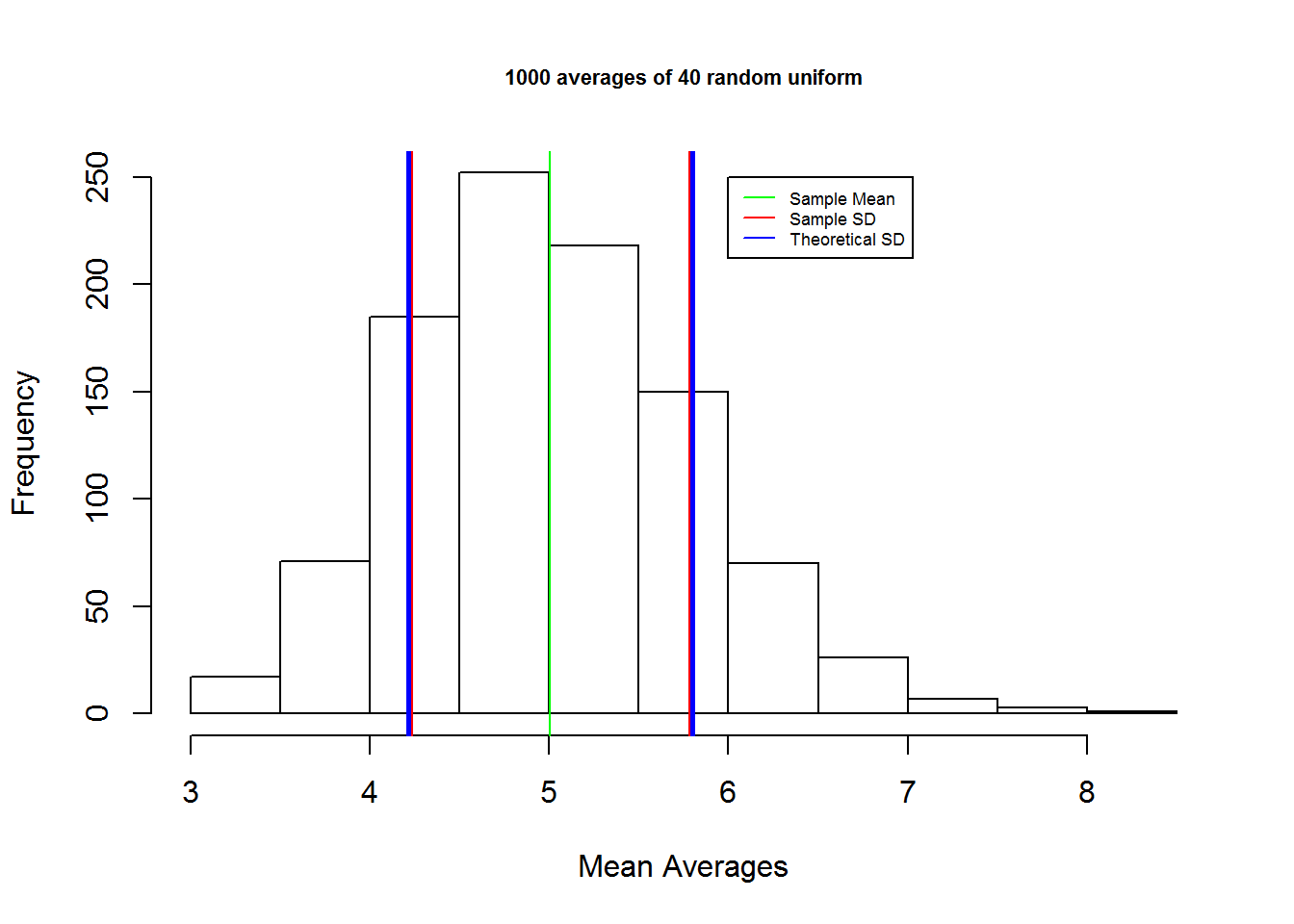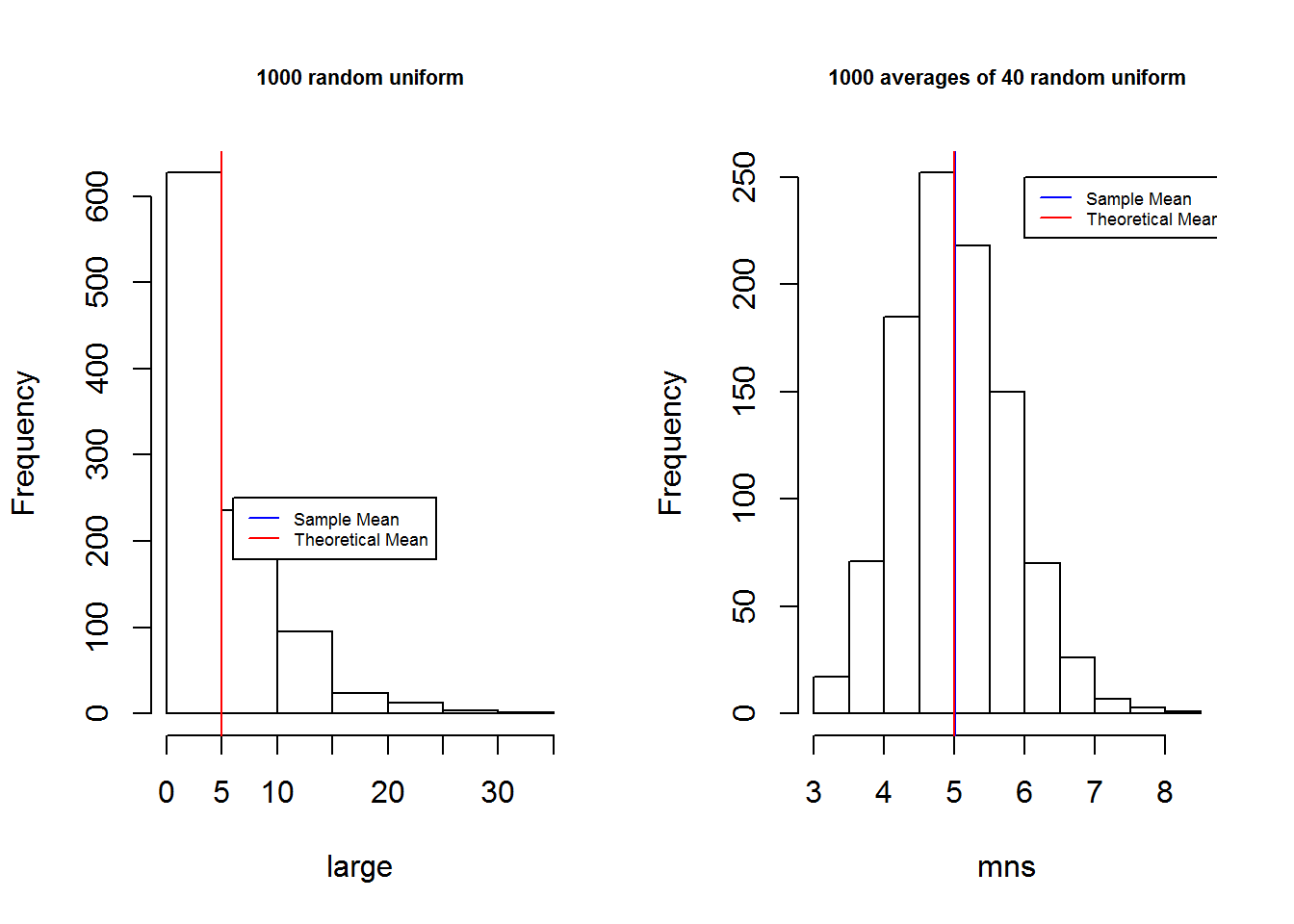### Comparing Exponential Distribution With Central Limit Theorem

Some notes based on my statistical studies. A good reference on how do simulations in R to prove and gain better grasp in understanding the Central Limit Theorem (CLT), and how CLT can even be used when your original data is not a normal distribution.

Or maybe perhaps you just prefer the video - Understanding Central Limit Theorem - with Bunnies and Dragons

### Overview

In this report we will investigate the exponential distribution in R and compare it with the Central Limit Theorem.

### Simulations

The exponential distribution will be simulated in R using rexp(n,lambda) where lambda is the rate parameter. The mean of exponential distribution is 1/lambda and the standard deviation is also 1/lambda. We will use lambda = 0.2 for all of the simulations.
We will illustrate via simulation and associated explanatory text the properties of the distribution of the mean of 40 exponentials. Among our findings would be:
1. The sample mean and it’s comparison to the theoretical mean of the distribution.
2. The sample variance and it’s comparison to the theoretical variance of the distribution.
3. The distribution and it’s normality.
Before we begin, let’s jot down some facts:
• The mean of the exponential distribution = 1/0.2 = 5.
• The standard deviation of the exponential distribution = 1/0.2 = 5.
• The sample size = 40.
• The simulation will be run 1000 times.

### Sample Mean versus Theoretical Mean

We start by illustrating the sample mean
``````# set seed so that our randomly generated graph remains the same for each run.
set.seed(10000)

#generate a sample of size 40 and iterate 1000 times.

mns = NULL
for (i in 1 : 1000) mns = c(mns, mean(rexp(40,rate=0.2)))

hist(mns, main="1000 averages of 40 random uniform", cex.main = 0.7)
abline(v=mean(mns),col="green")
abline(v=5,col="red")

legend( 6, 250, c("Sample Mean","Theoretical Mean"),lty=c(1,1),lwd=c(1,1,1),col=c("green","red"), cex = 0.55)````````````#find out the mean
mean(mns)``````
``##  5.00599``
As can be seen, the mean of the sample is 5.01. This is very close to the theoretical mean, 5.0.

### Sample Variance versus Theoretical Variance

Now we study the variability of the sample.
Considering the sample we’ve used previously, let’s find the variance. Or rather, let’s find the standard deviation for the graph since we know that standard deviation is basically just the square root of the variance - thus it should be sufficient in our attempt to prove the variability of the variance.
``````#get the standard deviation
#sd(mns)
hist(mns, main="1000 averages of 40 random uniform", cex.main = 0.7
, xlab="Mean Averages")
abline(v=mean(mns),col="green")
abline(v=mean(mns)+sd(mns), col="red", lwd=2.5)
abline(v=mean(mns)-sd(mns), col="red", lwd=2.5)

#now add the theoretical standard deviation
abline(v=mean(mns)+sqrt(5^2/40), col="blue", lwd=2.5)
abline(v=mean(mns)-sqrt(5^2/40), col="blue", lwd=2.5)

legend( 6, 250, c("Sample Mean","Sample SD","Theoretical SD"),lty=c(1,1),lwd=c(1,1,1),col=c("green","red","blue"), cex = 0.55)``````Based on the above the graph, we see that the sample standard deviation is very close to the theoretical standard deviation.
Thus we conclude that the variance is approximately similar.

### Distribution and Conclusion

Now let’s compare the distribution of a large collection of random exponentials (let say the size is 1000) against a distribution of a large collection of averages of 40 exponentials (let us say the collection size is 1000).
``````#generate the large sample of size 1000
large <- span=""> rexp(1000, rate=0.2)

mean(large)``````
``##  4.977901``
``````par(mfrow=c(1,2))
hist(large, main="1000 random uniform", cex.main = 0.7)
abline(v=mean(large),col="blue")
abline(v=5,col="red")
legend( 6, 250, c("Sample Mean","Theoretical Mean"),lty=c(1,1),lwd=c(1,1,1),col=c("blue","red"), cex = 0.55)

mean(mns)``````
``##  5.00599``
``````hist(mns, main="1000 averages of 40 random uniform", cex.main = 0.7)
abline(v=mean(mns),col="blue")
abline(v=5,col="red")
legend( 6, 250, c("Sample Mean","Theoretical Mean"),lty=c(1,1),lwd=c(1,1,1),col=c("blue","red"), cex = 0.55)``````Based on the simulation above, the mean for the first graph turned out to be 4.978, while the mean for the second graph was 5.01. Both values are really close the theoretical mean.
Looking at the histograms we see that the right histogram (average over sample) is more gaussian looking compared to the one the left.
We see that by taking repeated average many times across many small samples, we were able to derive a more accurate mean result.
In conclusion, the central limit theorem stipulates that a computed values of the average will be distributed accordingly to the normal distribution. Since our graph looks like a bell curve - we can claim that our graph approximately obeys the normal distribution.